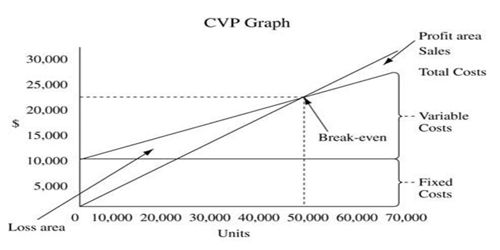# Different techniques applied for CVP Analysis

Different techniques applied for CVP Analysis

Cost Volume Profit (CVP) analysis is a managerial accounting technique that is concerned with the effect of sales volume and product costs on operating profit of a business. CVP analysis is the relationship among cost, volume, and profit when output increases units cost of production decrease vice versa. It deals with how operating profit is affected by changes in variable costs, fixed costs, selling price per unit and the sales mix of two or more different products. It is a powerful tool that helps managers understands the relationships of cost volume and profit.

CVP Analysis Formula

Profit equation: The basic formula used in CVP Analysis:

px = vx + FC + Profit

In the above formula,

• p is the price per unit;
• v is a variable cost per unit,
• x is the total number of units produced and sold; and,
• FC is the total fixed cost.

Besides the above formula, CVP analysis also makes use of the following concepts:

Contribution Margin (CM) – Contribution Margin (CM) is equal to the difference between total sales (S) and total variable cost or, in other words, it is the amount by which sales exceed total variable costs (VC). In order to make a profit the contribution margin of a business must exceed its total fixed costs.

In short: CM = S – VC

Unit Contribution Margin (Unit CM) – Contribution Margin can also be calculated per unit which is called Unit Contribution Margin. It is the excess of sales price per unit (p) over variable cost per unit (v). Thus: Unit CM = p – v.

Contribution Margin Ratio (CM Ratio) – Contribution Margin Ratio is calculated by dividing the contribution margin by total sales or unit CM by price per unit.

The contribution margin of an item is the difference between its sales price and the variable costs incurred to produce the product. This difference (the contribution margin) is at first used to cover fixed expenses: once those are covered, the company starts making a profit on the product(s).